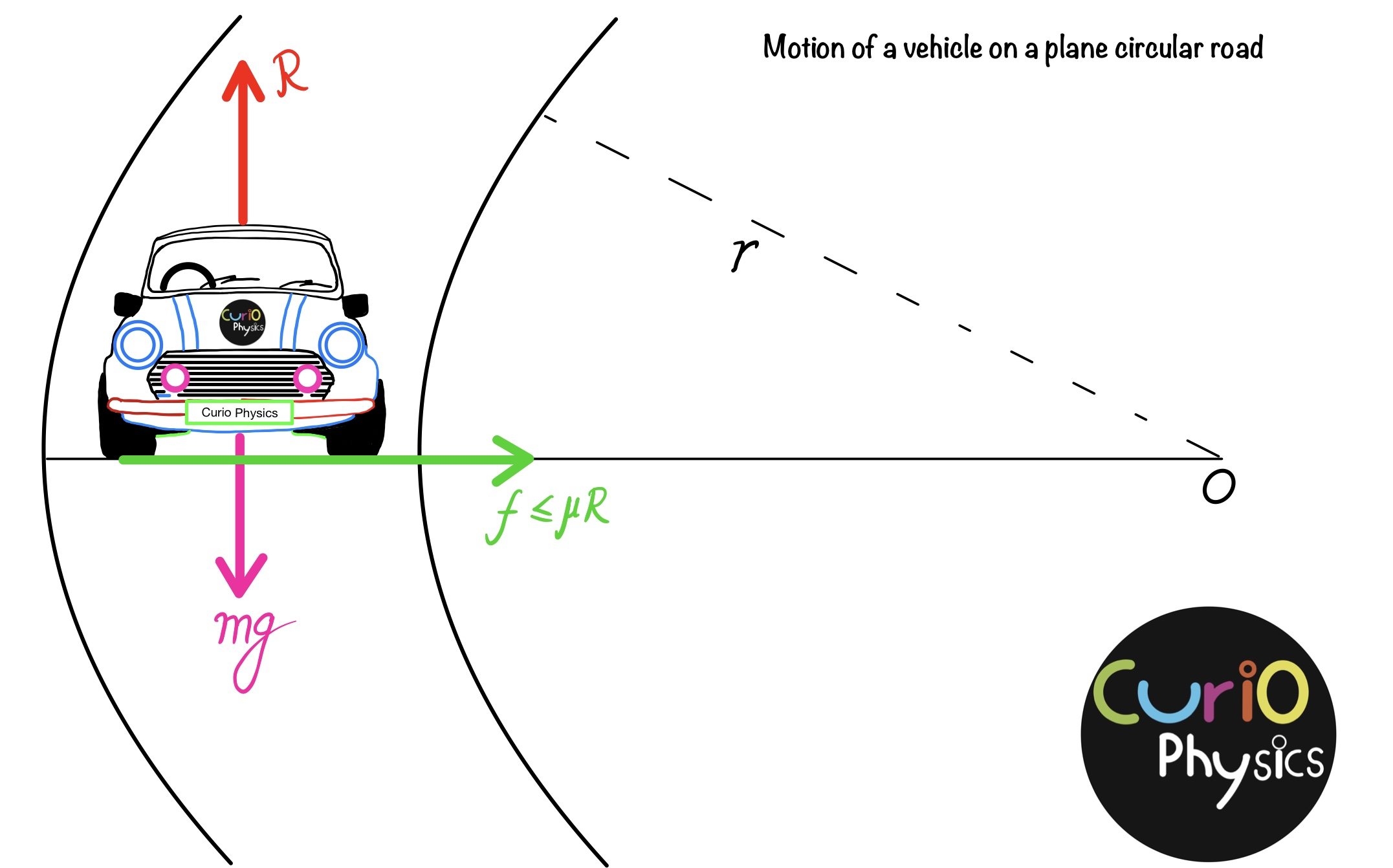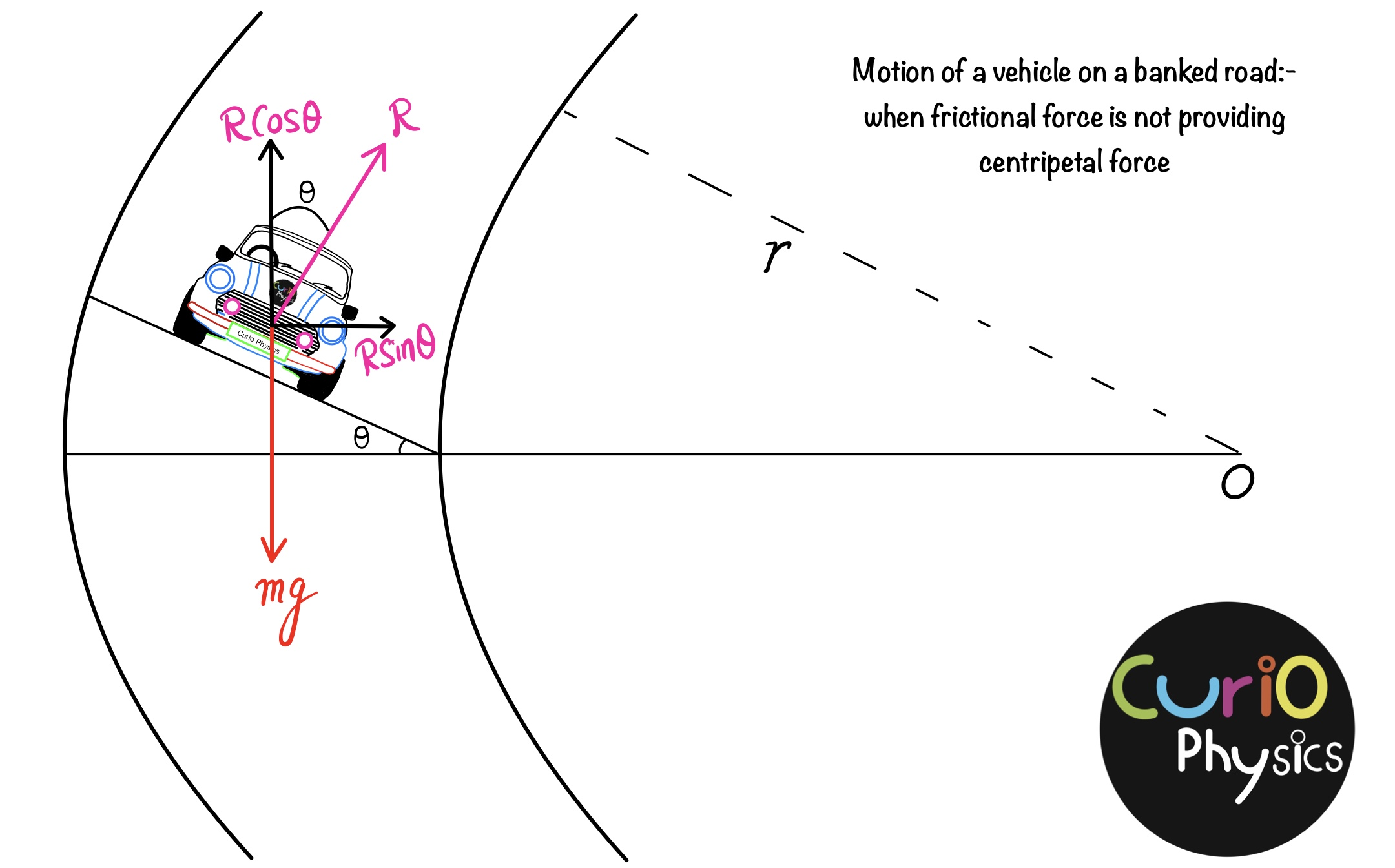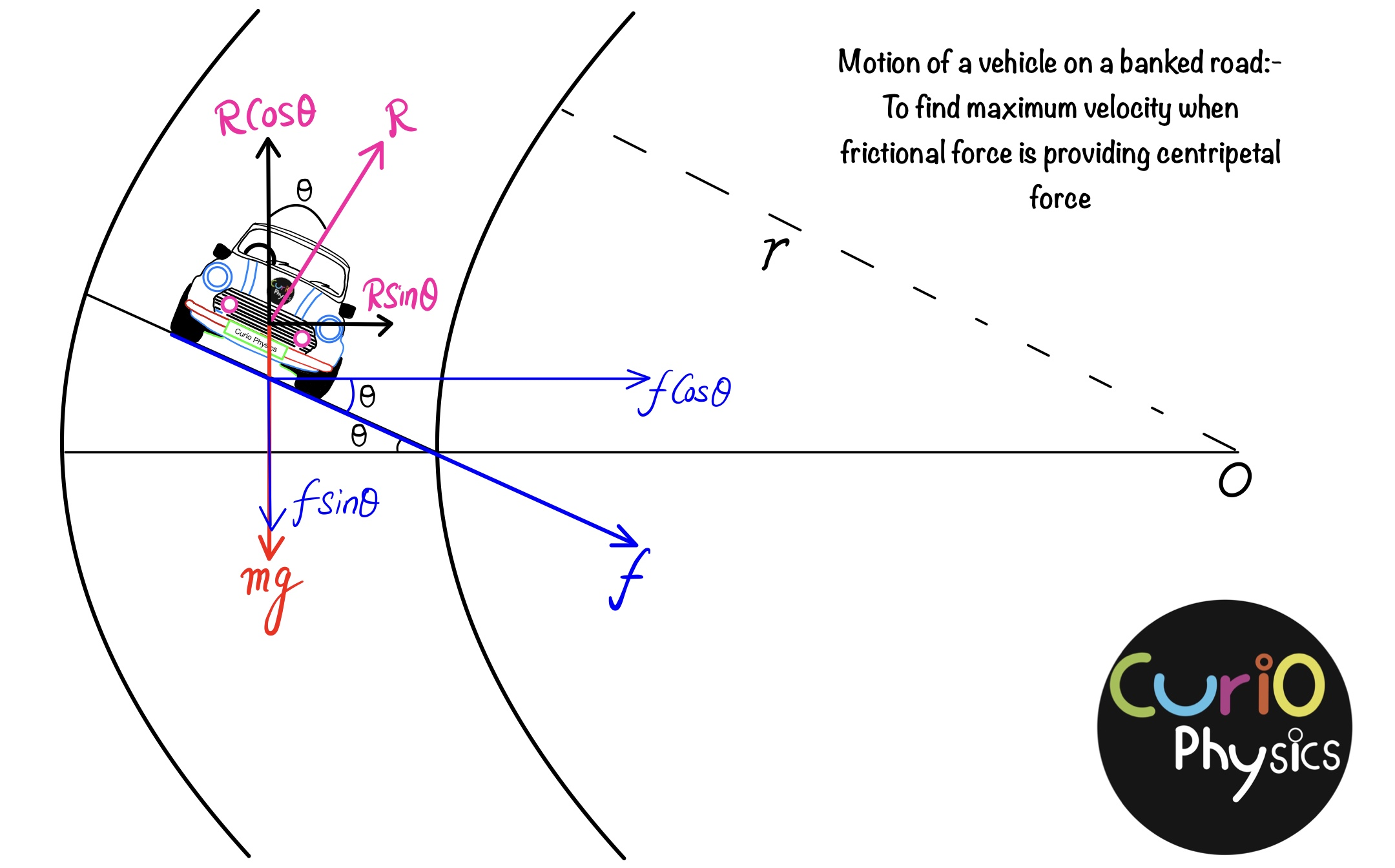# Motion of a vehicle on a plane and banked circular path

#### Motion of a vehicle on a plane and banked circular path

The vehicles require centripetal force at the bend of a road and this centripetal force is provided to the vehicle by the frictional force between the road and the tires of the vehicle or the radial component of the normal reaction on the vehicle by the road (when there is banking of the road) or by both during motion of a vehicle on a plane and banked circular path.

(1) Motion of a vehicle on a plan circular road

Suppose a car of mass m is moving with a fixed speed v on a flat circular road of radius r. Forces acting on the car: –

1. Car weight mg vertical downward.
2. Perpendicular reaction given by the road to the car  i.e., R vertically upward.
3. The friction force on the tires of the car, which is towards the center of the circular path (on a circular turn the car tends to leave the circular path and move away from the center of the turn so the friction force on the tires prevents the car from sliding ).

Here the frictional force f on the tires of the car provides the necessary centripetal force to move the car on a circular path and prevent the car from moving away from the center of the circular path.If the coefficient of friction between the tires and the road of the car is µ: –

Frictional force  f ≤ µR

By balancing the forces in the vertical direction: –

mg = R

Because the force of friction provides the necessary centripetal force to the car, so$\displaystyle f=\frac{m{{v}^{2}}}{r}$

For safe movement of the car on a flat circular path, the value of the centripetal force must be equal to or less then the force of friction.

So$\displaystyle \frac{m{{v}^{2}}}{r}\le \mu mg$$\displaystyle \Rightarrow v\le \sqrt{\mu rg}$

Hence the maximum safe speed of the car$\displaystyle {{v}_{\max }}=\sqrt{\mu rg}$

Note: – The maximum safe speed of the car does not depend on the mass of the car.

(2)  Motion of a vehicle on a banked road

Here 3 cases are possible :-

(a) When the frictional force is not providing the centripetal force : –

Let us suppose that the angle of banking of the road is θ and the radius of the curved road is r.From figure–$\displaystyle mg=R\cos \theta$……….(1)$\displaystyle \frac{m{{v}^{2}}}{r}=R\sin \theta$……….(2)

Dividing equation (2) by (1) —$\displaystyle \frac{m{{v}^{2}}}{rmg}=\frac{R\sin \theta }{R\cos \theta }$$\displaystyle \Rightarrow \frac{{{v}^{2}}}{rg}=\tan \theta$$\displaystyle \Rightarrow {{v}^{2}}=rg\tan \theta$$\displaystyle \Rightarrow v=\sqrt{rg\tan \theta }$……….(3)

Note: – With this speed, the wear of the tires of the car is reduced when driving the car on the sloping road.

(b) Maximum safe speed ( when frictional force is also providing centripetal force ) :-From figure —$\displaystyle mg+f\sin \theta =R\cos \theta$$\displaystyle \Rightarrow mg=R\cos \theta -f\sin \theta$……….(4)$\displaystyle \frac{m{{v}^{2}}}{r}=R\sin \theta +f\cos \theta$……….(5)

Dividing equation (5) by (4) —$\displaystyle \frac{m{{v}^{2}}}{rmg}=\frac{R\sin \theta +f\cos \theta }{R\cos \theta -f\sin \theta }$

If the maximum safe speed of the car us vmax , then$\displaystyle \frac{v_{\max }^{2}}{rg}=\frac{R\sin \theta +\mu R\cos \theta }{R\cos \theta -\mu R\sin \theta }$$\displaystyle \because f=\mu R$

Where the maximum value of frictional force is f  = μR$\displaystyle \frac{v_{\max }^{2}}{rg}=\frac{R\tan \theta +\mu R}{R-\mu R\tan \theta }=\frac{\tan \theta +\mu }{1-\mu \tan \theta }$$\displaystyle \Rightarrow v_{\max }^{2}=rg\left[ \frac{\tan \theta +\mu }{1-\mu \tan \theta } \right]$$\displaystyle \Rightarrow {{v}_{\max }}=\sqrt{rg\left[ \frac{\tan \theta +\mu }{1-\mu \tan \theta } \right]}$……….(6)

The maximum velocity of the car on the curved road is more than the ( ($\displaystyle {{v}_{\max }}=\sqrt{\mu rg}$) maximum velocity of the car on the plane road.

(c) Minimum safe speed (when frictional force is active) :-When the velocity of the car is less than$\sqrt{rg\tan \theta }$ , then it is possible that the car will slip inwards.

Now in this case the frictional force acts outwards, on changing the sign of f in equations (4) and (5) we get —$\displaystyle \ mg=R\cos \theta +f\sin \theta$……….(7)$\displaystyle \frac{m{{v}^{2}}}{r}=R\sin \theta -f\cos \theta$……….(8)

Dividing equation (8) by (7) —$\displaystyle \frac{m{{v}^{2}}}{rmg}=\frac{R\sin \theta -f\cos \theta }{R\cos \theta +f\sin \theta }$$\displaystyle \frac{{{v}^{2}}}{rg}=\frac{R\tan \theta -f }{R+f\tan\theta }$

When the velocity of the car is minimum, then the frictional force is maximum, i.e., f = μR.$\displaystyle \frac{v_{\min }^{2}}{rg}=\frac{R\tan \theta -\mu R}{R+\mu R\tan \theta }=\frac{\tan \theta -\mu }{1+\mu \tan \theta }$$\displaystyle \Rightarrow v_{\min }^{2}=rg\left[ \frac{\tan \theta -\mu }{1+\mu \tan \theta } \right]$$\displaystyle \Rightarrow {{v}_{\min }}=\sqrt{rg\left[ \frac{\tan \theta -\mu }{1+\mu \tan \theta } \right]}$……….(9)